JEE  >  Test: Single Correct MCQs: Quadratic Equation and Inequations (Inequalities) | JEE Advanced

# Test: Single Correct MCQs: Quadratic Equation and Inequations (Inequalities) | JEE Advanced

Test Description

## 39 Questions MCQ Test Mathematics For JEE | Test: Single Correct MCQs: Quadratic Equation and Inequations (Inequalities) | JEE Advanced

Test: Single Correct MCQs: Quadratic Equation and Inequations (Inequalities) | JEE Advanced for JEE 2023 is part of Mathematics For JEE preparation. The Test: Single Correct MCQs: Quadratic Equation and Inequations (Inequalities) | JEE Advanced questions and answers have been prepared according to the JEE exam syllabus.The Test: Single Correct MCQs: Quadratic Equation and Inequations (Inequalities) | JEE Advanced MCQs are made for JEE 2023 Exam. Find important definitions, questions, notes, meanings, examples, exercises, MCQs and online tests for Test: Single Correct MCQs: Quadratic Equation and Inequations (Inequalities) | JEE Advanced below.
 1 Crore+ students have signed up on EduRev. Have you?
Test: Single Correct MCQs: Quadratic Equation and Inequations (Inequalities) | JEE Advanced - Question 1

### If ℓ, m, n are real, ℓ ≠ m, then the roots by the equation: (ℓ – m)x2 – 5 (ℓ + m) x – 2 (ℓ – m) = 0 are (1979)

Detailed Solution for Test: Single Correct MCQs: Quadratic Equation and Inequations (Inequalities) | JEE Advanced - Question 1

ℓ, m, n are real, ℓ ≠ m

Given equation is

(ℓ - m) x 2 - 5(ℓ + m) x - 2(ℓ -m)= 0

D = 25(ℓ + m)2 + 8(ℓ - m)2 > 0,ℓ, m∈R

∴ Roots are real and unequal.

Test: Single Correct MCQs: Quadratic Equation and Inequations (Inequalities) | JEE Advanced - Question 2

### The equation x + 2y + 2z = 1 and 2x + 4y + 4z = 9 have

Detailed Solution for Test: Single Correct MCQs: Quadratic Equation and Inequations (Inequalities) | JEE Advanced - Question 2

The given equations are
x  + 2y + 2z = 1 ....(1)
and 2x + 4y + 4z = 9 ....(2)
Subtracting (1) × (2) from (2),
we get 0 =7 (not possible)
∴ No solution.

Test: Single Correct MCQs: Quadratic Equation and Inequations (Inequalities) | JEE Advanced - Question 3

### If x, y and z are real and different and (1979) u = x2 + 4y2 + 9z2 – 6yz – 3zx – 2xy, then u is always.

Detailed Solution for Test: Single Correct MCQs: Quadratic Equation and Inequations (Inequalities) | JEE Advanced - Question 3

u = x2 + 4y2 + 9z2 – 6yx – 3zx – 2xy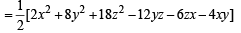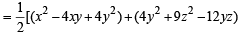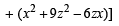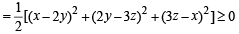∴ u is always non-negative.

Test: Single Correct MCQs: Quadratic Equation and Inequations (Inequalities) | JEE Advanced - Question 4

Let a > 0, b >0 and c > 0. Then the roots of the equation ax2 + bx + c = 0 (1979)

Detailed Solution for Test: Single Correct MCQs: Quadratic Equation and Inequations (Inequalities) | JEE Advanced - Question 4

As a, b, c > 0, a, b, c should be real (note that order relation is not defined in the set of complex numbers)
∴ Roots of equation are either real or complex conjugate.
Let α, β be the roots of ax2 +bx + c = 0, then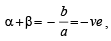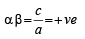⇒ Either both α, β are – ve (if roots are real) or both α, β have – ve real parts (if roots are complex conjugate)

Test: Single Correct MCQs: Quadratic Equation and Inequations (Inequalities) | JEE Advanced - Question 5

Both the roots of the equation (x – b) (x – c) + (x – a) (x – c) + (x – a) (x – b) = 0are always

Detailed Solution for Test: Single Correct MCQs: Quadratic Equation and Inequations (Inequalities) | JEE Advanced - Question 5

The given equation is (x -b)(x -c) + (x- a)(x - c) + (x - a)(x -b)= 0
⇒ 3x2 - 2(a+ b +c)x + (ab +bc +ca)=0

Discriminant = 4(a + b + c)2 – 12(ab + bc + ca)

= 4[a2 + b2 + c2 - ab - bc- ca]

= 2 [(a -b)2 +(b -c)2 +(c -a)2 ] ≥ 0 ∀ a, b, c

∴ Roots of given equation are always real.

Test: Single Correct MCQs: Quadratic Equation and Inequations (Inequalities) | JEE Advanced - Question 6

The least value of the expression 2 log10x – logx(0.01), for x > 1, is(1980)

Detailed Solution for Test: Single Correct MCQs: Quadratic Equation and Inequations (Inequalities) | JEE Advanced - Question 6

Let y = 2 log10 x – logx 0.01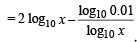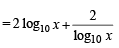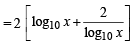[Here x  > 1 ⇒ log10 x > 0] Now since sum of a real + ve number and its reciprocal is always greater than or equal to 2.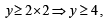∴ Least value of y is 4.

Test: Single Correct MCQs: Quadratic Equation and Inequations (Inequalities) | JEE Advanced - Question 7

If (x2 + px + 1) is a factor of (ax3 + bx + c), then (1980)

Detailed Solution for Test: Single Correct MCQs: Quadratic Equation and Inequations (Inequalities) | JEE Advanced - Question 7

As (x2 + px + 1) is a factor of ax3 + bx + c, we can assume that zeros of  x2 + px + 1 are a, b and that of ax3 + bx + c be α, β, γ so that

α + β = – p .... (i)

αβ = 1 .... (ii)

and  α + β + γ = 0 .... (iii)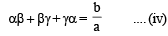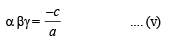Solving (ii) and (v) we get γ = – c / a.
Also from (i) and (iii) we get γ = p

∴ p = g = – c / a Using equations (i) , (ii) and (iv) we get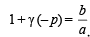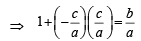(using  γ = p = – c / a)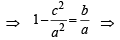a2 – c2= ab

∴ (c) is the  correct answer.

Test: Single Correct MCQs: Quadratic Equation and Inequations (Inequalities) | JEE Advanced - Question 8

The number  of real solutions of the equation | x |2 – 3 | x | + 2 = 0 is          (1982 - 2 Marks)

Detailed Solution for Test: Single Correct MCQs: Quadratic Equation and Inequations (Inequalities) | JEE Advanced - Question 8

| x |2 – 3 | x | + 2 = 0
Case  I   : x < 0 then | x | = – x
⇒ x2 + 3x + 2 = 0
⇒ (x + 1)  (x + 2) = 0 x = – 1, – 2 (both acceptable as < 0)
Case II: x > 0 then | x | = x
⇒ x2 – 3x + 2= 0
⇒ ( x – 1) (x – 2) = 0
x = 1, 2 ( both acceptable as > 0)
∴ There are 4 real solutions.

Test: Single Correct MCQs: Quadratic Equation and Inequations (Inequalities) | JEE Advanced - Question 9

Two towns A  and B are 60 km apart. A school is to be built to serve 150 students in town A and 50 students in town B. If the total distance to be travelled by all 200 students is to be as  small as possible, then the school should be built at (1982 - 2 Marks)

Detailed Solution for Test: Single Correct MCQs: Quadratic Equation and Inequations (Inequalities) | JEE Advanced - Question 9

Let the distance of school from A = x
∴ The distance of the school form B = 60 – x
Total distance covered by 200 students = 2[150 x + 50 (60 – x) ] = 2[100 x + 3000] This is min., when x = 0
∴ school should be built at town  A.

Test: Single Correct MCQs: Quadratic Equation and Inequations (Inequalities) | JEE Advanced - Question 10

If p, q, r are any real numbers, then (1982 - 2 Marks)

Detailed Solution for Test: Single Correct MCQs: Quadratic Equation and Inequations (Inequalities) | JEE Advanced - Question 10

if p = 5, q = 3, r = 2 max (p, q) = 5    ;    max (p, q, r) = 5
⇒ max (p, q) = max (p, q, r)
∴ (a) is not true. Similarly we can show that (c) is not true.

Also min (p,q) =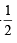(p + q- |q - p|)
Let p < q then LHS = p

and  R.H.S. =(p + q- q+ p) =p

Test: Single Correct MCQs: Quadratic Equation and Inequations (Inequalities) | JEE Advanced - Question 11

The largest interval for which x12 – x9 + x4 – x + 1 > 0 is           (1982 - 2 Marks)

Detailed Solution for Test: Single Correct MCQs: Quadratic Equation and Inequations (Inequalities) | JEE Advanced - Question 11

Given expression x12 - x9 + x4 - x + 1= f (x) (say)

For x < 0 put x = – y where y > 0

then we get f ( x) = y12 + y9 +y4 +y +1>0 for y > 0

For 0 < x < 1,   x9 < x 4 ⇒ - x9 +x> 0

Also  1– x  > 0 and x12 > 0

⇒ x12 – x9 + x4  + 1– x > 0    ⇒ f (x) > 0

For x > 1

f (x) = x (x3  – 1) (x8 + 1) + 1 > 0

So f (x) > 0 for –∞< x <∞

Test: Single Correct MCQs: Quadratic Equation and Inequations (Inequalities) | JEE Advanced - Question 12

The equation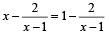has (1984 - 2 Marks)

Detailed Solution for Test: Single Correct MCQs: Quadratic Equation and Inequations (Inequalities) | JEE Advanced - Question 12

Given equation isClear ly x ≠ 1 for th e given eq. to be defin ed. If

x– 1 ≠ 0, we can cancel the common term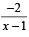on both sides to get x = 1, but it is not possible. So given eq. has no roots.

∴ (a) is the correct answer.

Test: Single Correct MCQs: Quadratic Equation and Inequations (Inequalities) | JEE Advanced - Question 13

If a2 + b2 + c2 = 1, then ab + bc + ca lies in the interval (1984 - 2 Marks)

Detailed Solution for Test: Single Correct MCQs: Quadratic Equation and Inequations (Inequalities) | JEE Advanced - Question 13

Given that a2 + b2 + c2 = 1 ....(1) We know (a + b +c)2  ≥ 0

⇒ a2 + b2 + c2 + 2ab + 2bc + 2ca ≥ 0

⇒ 2 ( ab + bc + c a ) ≥-1 [Using (1)]

⇒ ab + bc + ca ≥-1 / 2....(2) ....(2) Also we know that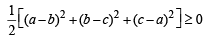Þ a2 + b2 + c2- ab- bc - ca≥ 0

Þ ab + bc + ca ≤ 1     [Using (1)]  .......(2)

Combining (2) and (3), we get

-1/ 2 ≤ ab + bc + ca≤1 ∴ab + bc + ca ∈ [-1/ 2,1]

∴ (c) is the correct answer.

Test: Single Correct MCQs: Quadratic Equation and Inequations (Inequalities) | JEE Advanced - Question 14

If log0.3 (x – 1) < log0.09(x – 1), then x  lies in the interval –           (1985 - 2 Marks)

Detailed Solution for Test: Single Correct MCQs: Quadratic Equation and Inequations (Inequalities) | JEE Advanced - Question 14

First of all for log (x – 1) to be defined, x – 1 > 0 ⇒ x  >  1 ....(1)
Now, log0.3 (x – 1) < log0.09  (x – 1)
⇒ log0.3 (x – 1) < log (0.3)2  (x – 1)
⇒ log0.3 (x – 1) <log0.3  (x – 1)
⇒ 2 log0.3 (x – 1) < log0.3  (x – 1)
⇒ log0.3 (x – 1)2 < log0.3  (x – 1)
⇒ (x – 1)2  >  (x – 1) NOTE THIS STEP
[The inequality is reversed since base lies between 0 and 1]
⇒ (x – 1)2  –  (x – 1) > 0 ⇒ (x – 1)  (x – 2) > 0  ....(2)
Combining (1) and (2) we get x  >  2
∴ x ∈ (2,∞)

Test: Single Correct MCQs: Quadratic Equation and Inequations (Inequalities) | JEE Advanced - Question 15

If α and β are the roots of x2 + px + q = 0 and α4, β4 are the roots of x2 – rx + s = 0, then the equation x2 – 4qx + 2q2 – r = 0 has always (1989 - 2 Marks)

Detailed Solution for Test: Single Correct MCQs: Quadratic Equation and Inequations (Inequalities) | JEE Advanced - Question 15

From the first method,

q = αβ, r = α44 ...(3)

Product of the roots of the equation x2 – 4qx + (2q2 – r) = 0

= 2q2 – r = 2α2β2 – α4 – β4 = – (α2 – β2)2 [From (3)]

= – (positive quantity) = – ve quantity
⇒  one root is positive and other is negative

Test: Single Correct MCQs: Quadratic Equation and Inequations (Inequalities) | JEE Advanced - Question 16

Let a, b, c be real numbers, a ≠ 0. If α is a r oot of a2x2 + bx + c = 0 . β is the root of a2x2 – bx – c = 0 and 0 < α < β, then the equation a2x2 + 2bx + 2c = 0 has a root g that always satisfies (1989 - 2 Marks)

Detailed Solution for Test: Single Correct MCQs: Quadratic Equation and Inequations (Inequalities) | JEE Advanced - Question 16

KEY CONCEPT : If f (α) and f (β) are of opposite signs then there must lie a value γ between α and β such that f (γ) = 0.
a, b, c are real numbers and a ≠ 0.
As α is a root of a2x2 + bx + c = 0
∴ a2α2 + bα + c = 0 ....(1)
Also b is a root of a2x2 – bx – c = 0  ∴
a2β2 – bβ –  c = 0 .... (2)
Now, let f (x) = a2x2 + 2bx + 2c
Then f (α) = a2α2  + 2bα + 2c = a2α2 + 2(bα + c)
= a2α2 + 2(– a2α2) [Using eq. (1)]
= – a2α2.
and f (β) = a2β2 + 2bβ + 2c
= a2β2 + 2(bβ + c)
= a2β2 + 2(a2β2) [Using eq. (2)]
= 3a2β2 > 0.
Since f (α) and f (β) are of opposite signs and γ is a root of equation f (x) = 0
∴ γ must lie between α and β
Thus α < γ < β.     ∴    (d) is the correct option.

Test: Single Correct MCQs: Quadratic Equation and Inequations (Inequalities) | JEE Advanced - Question 17

The number of solutions of the equation sin(e)x = 5x + 5–x is            (1990 -  2 Marks)

Detailed Solution for Test: Single Correct MCQs: Quadratic Equation and Inequations (Inequalities) | JEE Advanced - Question 17

The given eq. is sin (ex) = 5x + 5–x We know 5x and 5–x both are +ve real numbers using

AM ≥ GM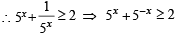∴ R.H.S. of given eq. ≥ 2 While sin ex ∈ [-1,1] i . e . LHS ∈ [-1,1]
∴ The equation is not possible for any real value of x.
Hence (a) is the correct answer.

Test: Single Correct MCQs: Quadratic Equation and Inequations (Inequalities) | JEE Advanced - Question 18

Let α, β be the roots of the equation (x – a) (x – b) = c, c ≠ 0.Then the roots of the equation (x – α) (x – β) + c = 0 are (1992 -  2 Marks)

Detailed Solution for Test: Single Correct MCQs: Quadratic Equation and Inequations (Inequalities) | JEE Advanced - Question 18

α, β are roots of the equation (x – a) (x – b) = c, c ≠ 0
∴ (x – a) (x – b) – c = (x – α)(x – β)
⇒ (x – α) (x – β) + c = (x – a)(x – b)
⇒ roots of (x – α) (x – β) + c = 0 are α and β.
∴ (c) is the correct option.

Test: Single Correct MCQs: Quadratic Equation and Inequations (Inequalities) | JEE Advanced - Question 19

The number of poin ts of intersection of two curves y = 2 sinx and y = 5x2 + 2x + 3 is (1994)

Detailed Solution for Test: Single Correct MCQs: Quadratic Equation and Inequations (Inequalities) | JEE Advanced - Question 19

We have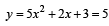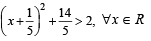while y =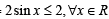⇒ The two curves do not meet at all.

Test: Single Correct MCQs: Quadratic Equation and Inequations (Inequalities) | JEE Advanced - Question 20

If p, q, r are +ve and are in A.P., the roots of quadratic equation px2 + qx + r = 0 are all real for (1994)

Detailed Solution for Test: Single Correct MCQs: Quadratic Equation and Inequations (Inequalities) | JEE Advanced - Question 20

For real roots q2 - 4 pr≥0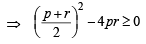(∵ p,  q, r are in A.P.)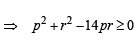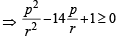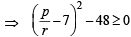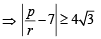Test: Single Correct MCQs: Quadratic Equation and Inequations (Inequalities) | JEE Advanced - Question 21

Let p,q ∈ {1,2, 3,4} . The number of equations of the form px2 + qx + 1 = 0 having real roots is (1994)

Detailed Solution for Test: Single Correct MCQs: Quadratic Equation and Inequations (Inequalities) | JEE Advanced - Question 21

For the equation px2 + qx + 1 = 0 to have real roots

D ≥ 0 ⇒ q2 ≥ 4p

If  p = 1 then  q 2 ≥ 4 ⇒ q = 2, 3, 4

If  p = 2 then  q 2 ≥ 8 ⇒ q = 3, 4

If  p = 3 then  q2 ≥ 12 ⇒ q =4

If  p = 4 then  q2 ≥ 16 ⇒ q = 4

∴ No. of req. equations = 7.

Test: Single Correct MCQs: Quadratic Equation and Inequations (Inequalities) | JEE Advanced - Question 22

If the roots of the equation x2 – 2ax + a2 + a – 3 = 0 are real and less than 3, then (1999 - 2 Marks)

Detailed Solution for Test: Single Correct MCQs: Quadratic Equation and Inequations (Inequalities) | JEE Advanced - Question 22

KEY CONCEPT : If both roots of a quadratic equation ax2 + bx + c = 0 are less than k then af(k)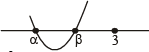> 0, D ≥ 0, α + β < 2 k.

f(x) = x2 – 2ax + a2 + a – 3 = 0,

f(3) > 0, α + β < 6, D ≥ 0

⇒ a2 – 5a + 6 > 0, a < 3, – 4a + 12 ≥ 0

⇒ a < 2 or a > 3, a < 3, a < 3  ⇒ a < 2.

Test: Single Correct MCQs: Quadratic Equation and Inequations (Inequalities) | JEE Advanced - Question 23

If α and β (α < β) are the roots of the equation x2 + bx + c = 0, where c < 0 < β, then (2000S)

Detailed Solution for Test: Single Correct MCQs: Quadratic Equation and Inequations (Inequalities) | JEE Advanced - Question 23

Given c < 0 < b   and α + β = – b ....(1) αβ = c
....(2)

From (2), c < 0 ⇒ αβ < 0 ⇒ either a is  -ve or β is - ve and second ;quantity is positive.

from (1), b > 0 ⇒ – b < 0  ⇒ α + β < 0 ⇒ the sum is negative

⇒ modules of nengative quantity is > modulus of positive quantity but α< β is given.
Therefore, it is clear that α is negative and β is positive and modulus of α is greater than modulus of β ⇒ α< 0 <β< |α|

Test: Single Correct MCQs: Quadratic Equation and Inequations (Inequalities) | JEE Advanced - Question 24

If a, b, c, d are positive real numbers such that a + b + c + d = 2, then M = (a + b) (c + d) satisfies the relation

Detailed Solution for Test: Single Correct MCQs: Quadratic Equation and Inequations (Inequalities) | JEE Advanced - Question 24

As A.M. ≥ G.M.  for positive real numbers,  we get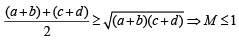(Putting values)

Also (a + b) (c + d) > 0             [∴    a,b,c,d > 0]

∴ 0 ≤ M≤1

Test: Single Correct MCQs: Quadratic Equation and Inequations (Inequalities) | JEE Advanced - Question 25

If b > a, then the equation (x – a) (x – b) –1 = 0 has (2000S)

Detailed Solution for Test: Single Correct MCQs: Quadratic Equation and Inequations (Inequalities) | JEE Advanced - Question 25

The given equation is ( x – a) (x – b) – 1 = 0, b > a.

or x2 – (a + b) x + ab –1= 0

Let  f(x) = x2 – (a + b) x + ab – 1

Since coeff. of x2 i.e. 1 > 0,
∴ it represents upward parabola, intersecting x - axis at two points. (corresponding to two real roots, D being +ve).

Also f (a) = f (b) = – 1 ⇒ curve is below x-axis at a and b

⇒ a and b both lie between the roots.
Thus the graph of given eqn is as shown.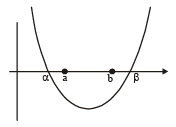from graph it is clear that one root of the equation lies in (– ∞, a) and other in (b, ∞).

Test: Single Correct MCQs: Quadratic Equation and Inequations (Inequalities) | JEE Advanced - Question 26

For the equation 3x2 + px + 3 = 0, p > 0, if one of the root is square of the other, then p is equal to (2000S)

Detailed Solution for Test: Single Correct MCQs: Quadratic Equation and Inequations (Inequalities) | JEE Advanced - Question 26

Let α, α2 be the roots of  3x2 + px + 3.

∴ α + α2 = – p / 3 and α3 = 1

⇒ (α – 1) (α2 + α + 1) = 0 ⇒ α =1 or  α2 + α  = – 1

If α = 1, p = – 6 which is not possible as p > 0

If α2 + α = –1  ⇒ – p/3= –1 ⇒ p = 3.

Test: Single Correct MCQs: Quadratic Equation and Inequations (Inequalities) | JEE Advanced - Question 27

If a1,a2.....,an are positive real numbers whose product is a fixed number c, then the minimum value of a1 + a2 + .......+an-1 + 2an is (2002S)

Detailed Solution for Test: Single Correct MCQs: Quadratic Equation and Inequations (Inequalities) | JEE Advanced - Question 27

We have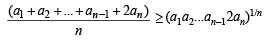[Using A.M. ≥ G.M.]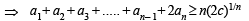Test: Single Correct MCQs: Quadratic Equation and Inequations (Inequalities) | JEE Advanced - Question 28

The set of all real numbers x for which x2 – | x + 2 | + x > 0, is (2002S)

Detailed Solution for Test: Single Correct MCQs: Quadratic Equation and Inequations (Inequalities) | JEE Advanced - Question 28

For x < – 2 ,   | x + 2 | = – (x + 2) and the  inequality becomes

x2 + x + 2 + x  > 0     ⇒ (x + 1)2  + 1 > 0

which is valid ∀ x∈R but x < – 2

∴ x ∈ ( - ∞,- 2) ....(1)

For x ≥ 2, | x + 2 |=x+2 and the inequality becomes

x2  – x – 2 + x > 0 ⇒ x2 > 2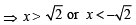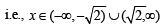but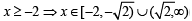....(2)

From (1) and (2)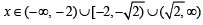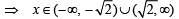Test: Single Correct MCQs: Quadratic Equation and Inequations (Inequalities) | JEE Advanced - Question 29

If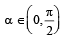then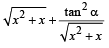is always greater than or equal to (2003S)

Detailed Solution for Test: Single Correct MCQs: Quadratic Equation and Inequations (Inequalities) | JEE Advanced - Question 29

Let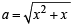and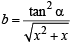then using AM GM, we get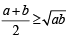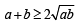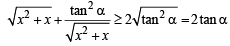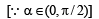Test: Single Correct MCQs: Quadratic Equation and Inequations (Inequalities) | JEE Advanced - Question 30

For all ‘x’, x2 + 2ax + 10– 3a > 0, then the interval in which ‘a’ lies is (2004S)

Detailed Solution for Test: Single Correct MCQs: Quadratic Equation and Inequations (Inequalities) | JEE Advanced - Question 30

KEY CONCEPT : f(x) = ax2 + bx + c has same sign as that of a if  D < 0.

x2 + 2ax + 10 - 3a >0∀x

⇒ D < 0 ⇒ 4a2 - 4(10 - 3a)< 0 ⇒ a2 + 3a - 10<0

⇒ (a + 5)(a - 2) < 0 ⇒a ∈ (-5, 2)

Test: Single Correct MCQs: Quadratic Equation and Inequations (Inequalities) | JEE Advanced - Question 31

If one root is square of the oth er root of the equation x2 + px + q = 0, then the relation between p and q is  (2004S)

Detailed Solution for Test: Single Correct MCQs: Quadratic Equation and Inequations (Inequalities) | JEE Advanced - Question 31

x2 + px +q= 0

Let roots be a and a2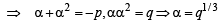∴ (q)1/3 + (q1/3)2 =-p

Taking cube of both sides, we get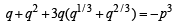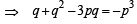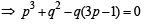Test: Single Correct MCQs: Quadratic Equation and Inequations (Inequalities) | JEE Advanced - Question 32

Let a, b, c be the sides of a triangle where a ≠ b ≠ c and λ ∈ R. If the roots of the equation x2 + 2(a + b + c)x + 3λ (ab + bc + ca) = 0 are real, then (2006 - 3M, –1)

Detailed Solution for Test: Single Correct MCQs: Quadratic Equation and Inequations (Inequalities) | JEE Advanced - Question 32

∵ a, b, c are sides of a triangle and a ≠ b≠c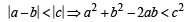Similarly, we have

b2 + c2 - 2bc < a2 ; c2 + a2 -2ca<b2

a2 + b2 + c2 < 2(ab + bc+ ca)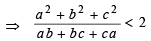....(1)

∵ Roots of the given equation are real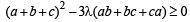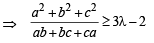....(2)

From (1) and (2), we get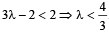Test: Single Correct MCQs: Quadratic Equation and Inequations (Inequalities) | JEE Advanced - Question 33

Let α, β be the roots of the equation x2 – px + r = 0 and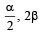be the roots of the equation x2 – qx + r = 0. Then the value of r is (2007 -3 marks)

Detailed Solution for Test: Single Correct MCQs: Quadratic Equation and Inequations (Inequalities) | JEE Advanced - Question 33

As α, β are the roots of  x2 – px + r = 0

∴ α + β = p   ....(1)

and αβ = r   ....(2)

Also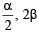are the roots of  x2 – qx + r = 0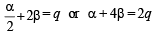....(3)

Solving (1) and (3) for α and β ,we get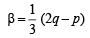and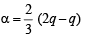Substituting values of α and β, in equation (2),

we get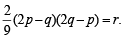Test: Single Correct MCQs: Quadratic Equation and Inequations (Inequalities) | JEE Advanced - Question 34

Let p and q be real numbers such that p ≠ 0, p≠q and p3 ≠-q . If a and b are nonzero complex numbers satisfying α + β = – p and α3 + β3 = q, then a quadratic equation having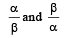as its roots is (2010)

Detailed Solution for Test: Single Correct MCQs: Quadratic Equation and Inequations (Inequalities) | JEE Advanced - Question 34

Given that α + β= – p and α3 + β3= q

⇒ (α + β)3 – 3αβ (α + β)= q

⇒ – p3 – 3αβ (– p)=q ⇒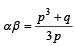sum of roots =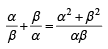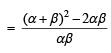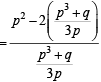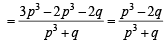and product of roots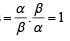∴ Required equation is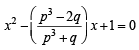or  ( p3 + q)x2 – ( p3 – 2q)x + ( p3 + q)= 0

Test: Single Correct MCQs: Quadratic Equation and Inequations (Inequalities) | JEE Advanced - Question 35

Let (x0, y0) be the solution of the following equations
(2x)ℓn2 = (3y)ℓn3
3ℓnx =2ℓny
Then x0 is (2011)

Detailed Solution for Test: Single Correct MCQs: Quadratic Equation and Inequations (Inequalities) | JEE Advanced - Question 35

We have (2x)ℓn2 = (3y)ℓn3
⇒ ℓn2. ℓn2x = ℓn3. ℓn3y
⇒ ℓn2. ℓn2x = ℓn3. (ℓn3 + ℓny) ...(1)
Also given 3ℓnx = 2lny

⇒ ℓnx. ℓn3 = ℓny. ℓn2 ⇒ ℓny =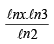Substituting this value of ℓny in equation (1), we get

ℓn2. ℓn2x = ℓn3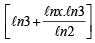⇒ (ℓn2)2 ℓn2x = (ℓn3)2 ℓn2 + (ℓn3)2 ℓnx

⇒ (ℓn2)2 ℓn2x = (ℓn3)2 (ℓn2 + ℓnx)

⇒ (ℓn2)2 ℓn2x – (ℓn3)2 ℓn2x = 0

⇒ [(ℓn2)2 – (ℓn3)2] ℓn2x = 0  ⇒ ℓn2x = 0

⇒ 2x = 1   or x =Test: Single Correct MCQs: Quadratic Equation and Inequations (Inequalities) | JEE Advanced - Question 36

Let α and β be the roots of x2 – 6x – 2 = 0, with α > β. If an = αn - βn for n ≥ 1, then the value ofis (2011)

Detailed Solution for Test: Single Correct MCQs: Quadratic Equation and Inequations (Inequalities) | JEE Advanced - Question 36

∵ α, β are the roots of x2 – 6x – 2 = 0

∴ α2 – 6α – 2 = 0

⇒ α10 – 6α9– 2α8 = 0

⇒ α10 – 2α8 = 6α9 ...(1)

Similarly β10 – 2β8  = 6β9 ...(2)

From equation (1) and (2

α10 – β10 – 2 (α8– β8) = 6 (α9– β9)

⇒ a10 – 2a8 = 6a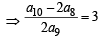Test: Single Correct MCQs: Quadratic Equation and Inequations (Inequalities) | JEE Advanced - Question 37

A value of b for which the equations
x2 + bx – 1 = 0
x2 + x + b = 0
have one root in common is (2011)

Detailed Solution for Test: Single Correct MCQs: Quadratic Equation and Inequations (Inequalities) | JEE Advanced - Question 37

Let α be the common root of given equations, then
α2 + bα – 1 = 0 ...(1)
and α2 + α + b = 0 ...(2)
Subtracting (2) from (1),
we get (b – 1) α – (b + 1) = 0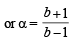Substituting this value of α in equation (1), we get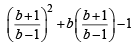= 0  or  b3 + 3b = 0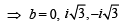Test: Single Correct MCQs: Quadratic Equation and Inequations (Inequalities) | JEE Advanced - Question 38

The quadratic equation p(x) = 0 with real coefficients has purely imaginary roots. Then the equation p(p(x)) = 0 has (JEE Adv. 2014)

Detailed Solution for Test: Single Correct MCQs: Quadratic Equation and Inequations (Inequalities) | JEE Advanced - Question 38

Quadratic equation with real coefficients and purely imaginary roots can be considered as

p(x) = x2 + a = 0 where a > 0 and a ∈R
The p[ p(x)] = 0 ⇒ (x2 + a)2 + a = 0
⇒ x4 + 2ax2 + (a2 + a) = 0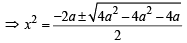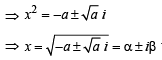where a, b≠0

∴ p[p(x)] = 0 has complex roots which are neither purely real nor purely imaginary.

Test: Single Correct MCQs: Quadratic Equation and Inequations (Inequalities) | JEE Advanced - Question 39

Let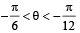Suppose α1 and B1are the roots of the equation x2 – 2x sec a + 1 = 0 and α2 and β2 are the roots of the equation x2 + 2x tanθ – 1 = 0. If α1 > b1 and α2 > β2, then α1 + β2 equals (JEE Adv. 2016)

Detailed Solution for Test: Single Correct MCQs: Quadratic Equation and Inequations (Inequalities) | JEE Advanced - Question 39

x2 – 2x secθ + 1 = 0 ⇒ x = secθ ± tanθ and x2 + 2x tanθ – 1 = 0 ⇒ x = –tanθ ± secθ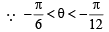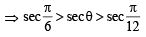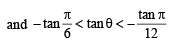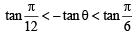α11 are roots of x2 – 2x secθ + 1 = 0 and α1> b1

∴ α1 = secθ – tanθ and b1 = secθ + tanθ α2,
β2 are roots of x2 + 2x tanθ – 1 = 0 and α2 > β2
∴ α2 = -tanθ + secθ, β2 = – tanθ – secθ
∴ α1 + β2 = secθ – tanθ – tanθ – secθ = – 2tanθ

## Mathematics For JEE

130 videos|359 docs|306 tests
 Use Code STAYHOME200 and get INR 200 additional OFF Use Coupon Code